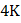# A child is standing with folded hands at the centre of a platform rotating about its central axis. The kinetic energy of the system is. The child stretches his arms so that the moment of inertia of the system doubles. The kinetic energy of the system now is a)b)c)d)## Question ID - 100212 :- A child is standing with folded hands at the centre of a platform rotating about its central axis. The kinetic energy of the system is. The child stretches his arms so that the moment of inertia of the system doubles. The kinetic energy of the system now is a)b)c)d)3537

(b)

From conservation of angular momentum (= constant), angular velocity will remain half. As,The rotational kinetic energy will become half.

Next Question :

A slender rod of massand lengthis pivoted about a horizontal axis through one end and released from rest at an angle of 30above the horizontal. The force exerted by the pivot on the rod are the instant when the rod passes through a horizontal position isa)along horizontal b)along vertical c)along a line making an angle ofwith the horizontal d)along a line making an angle ofwith the horizontal